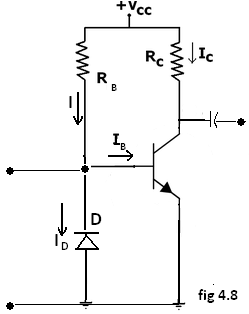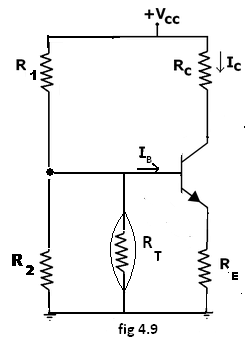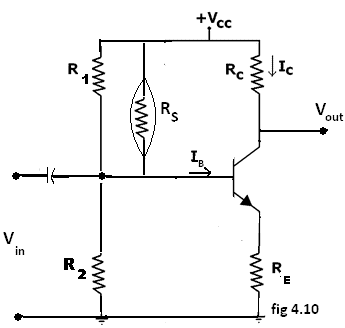Courses

# Bias Compensation Using Diode And Transistor Electrical Engineering (EE) Notes | EduRev

## Electronic Devices

Created by: Cstoppers Instructors

## Electrical Engineering (EE) : Bias Compensation Using Diode And Transistor Electrical Engineering (EE) Notes | EduRev

The document Bias Compensation Using Diode And Transistor Electrical Engineering (EE) Notes | EduRev is a part of the Electrical Engineering (EE) Course Electronic Devices.
All you need of Electrical Engineering (EE) at this link: Electrical Engineering (EE)

BIAS COMPENSATION USING DIODE AND TRANSISTOR:

DIODE COMPENSATION:The following fig 4.8 shows a transistor amplifier with a diode D connected across the base-emitter junction for compensation of change in collector saturation current ICO. The diode is of the same material as the transistor and it is reverse biased by e the emitter-base junction voltage VBE, allowing the diode reverse saturation current IO to flow through diode D. The base current IB=I-IO.

As long as temperature is constant, diode D operates as a resistor. As the temperature increases, ICO of the transistor increases. Hence, to compensate for this, the base current IB should be decreased.

The increase in temperature will also cause the leakage current IO through D to increase and thereby decrease the base current IB. This is the required action to keep Ic constant.

This type of bias compensation does not need a change in Ic to effect the change in IC, as both IO and ICO can track almost equally according to the change in temperature.

THERMISTOR COMPENSATION:

The following fig 4.9 a thermistor RT, having a negative temperature coefficient is connected in parallel with R2. The resistance of thermistor decreases exponentially with increase of temperature. An increase of temperature will decrease the base voltage VBE, reducing IB and IC.SENSISTOR COMPENSATION:

In the following fig 4.10 shown a sensistor Rs having a positive temperature coefficient is connected across R1 or RE. Rs increases with temperature. As the temperature increases, the equivalent resistance of the parallel combination of R1 and Rs also increases and hence VBE decreases, reducing Iand Ic. This reduced Ic compensates for increased Ic caused by the increase in VBE, ICO and β due to temperature.Offer running on EduRev: Apply code STAYHOME200 to get INR 200 off on our premium plan EduRev Infinity!

,

,

,

,

,

,

,

,

,

,

,

,

,

,

,

,

,

,

,

,

,

;